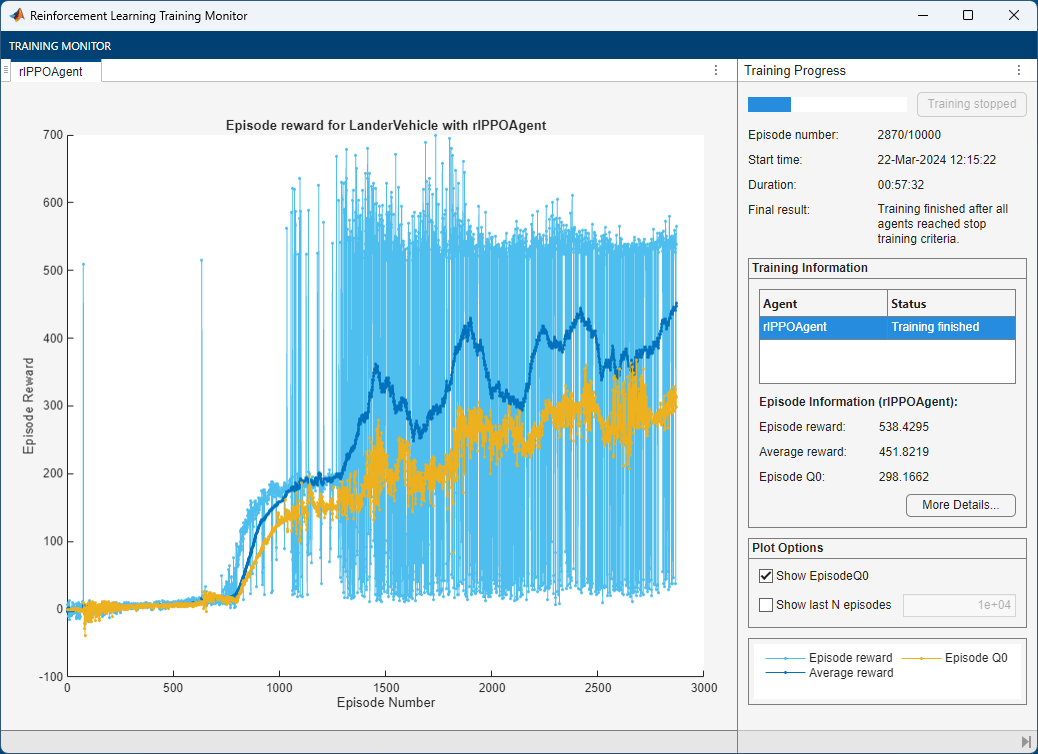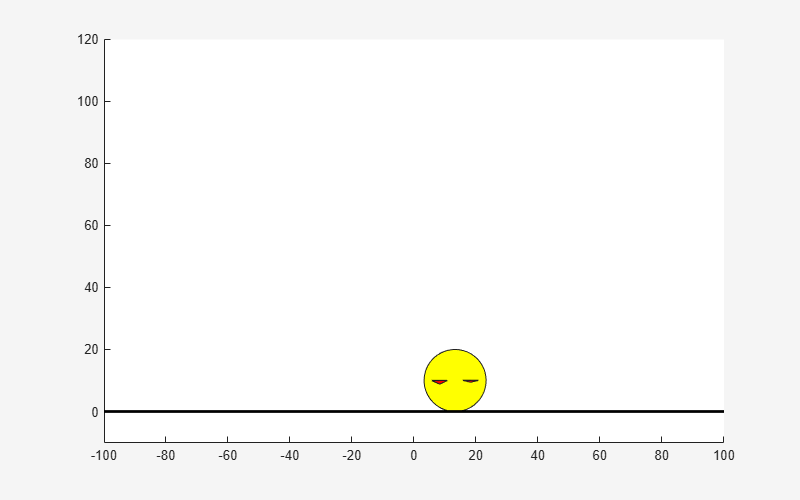# Train PPO Agent for a Lander Vehicle

This example shows how to train a proximal policy optimization (PPO) agent with a discrete action space to land an airborne vehicle on the ground. For more information on PPO agents, see Proximal Policy Optimization (PPO) Agents.### Environment

The environment in this example is a lander vehicle represented by a 3-DOF circular disc with mass. The vehicle has two thrusters for forward and rotational motion. Gravity acts vertically downwards, and there are no aerodynamic drag forces. The training goal is to make the vehicle land on the ground at a specified location.

For this environment:

• Motion of the lander vehicle is bounded in X (horizontal axis) from -100 to 100 meters and Y (vertical axis) from 0 to 120 meters.

• The goal position is at (0,0) meters and the goal orientation is 0 radians.

• The maximum thrust applied by each thruster is 8.5 N.

• The sample time is 0.1 seconds.

• The observations from the environment are the vehicle's position $\left(\mathit{x},\mathit{y}\right)$, orientation $\left(\theta \right)$, velocity $\left(\stackrel{˙}{\mathit{x}},\stackrel{˙}{\mathit{y}}\right)$, angular velocity $\left(\stackrel{˙}{\theta }\right)$, and a sensor reading that detects rough landing (-1), soft landing (1) or airborne (0) condition. The observations are normalized between -1 and 1.

• The environment has a discrete action space. At every time step, the agent selects one of the following nine discrete action pairs:

`$\begin{array}{l}\mathit{L},\text{\hspace{0.17em}}\mathit{L}-\mathrm{do}\text{\hspace{0.17em}}\mathrm{nothing}\\ \mathit{L},\text{\hspace{0.17em}}\mathit{M}-\mathrm{fire}\text{\hspace{0.17em}}\mathrm{right}\text{\hspace{0.17em}}\left(\mathrm{med}\right)\\ \mathit{L},\text{\hspace{0.17em}}\mathit{H}-\mathrm{fire}\text{\hspace{0.17em}}\mathrm{right}\text{\hspace{0.17em}}\left(\mathrm{high}\right)\\ \mathit{M},\text{\hspace{0.17em}}\mathit{L}-\mathrm{fire}\text{\hspace{0.17em}}\mathrm{left}\text{\hspace{0.17em}}\left(\mathrm{med}\right)\\ \mathit{M},\text{\hspace{0.17em}}\mathit{M}-\mathrm{fire}\text{\hspace{0.17em}}\mathrm{left}\text{\hspace{0.17em}}\left(\mathrm{med}\right)+\mathrm{right}\text{\hspace{0.17em}}\left(\mathrm{med}\right)\\ \mathit{M},\text{\hspace{0.17em}}\mathit{H}-\mathrm{fire}\text{\hspace{0.17em}}\mathrm{left}\text{\hspace{0.17em}}\left(\mathrm{med}\right)+\mathrm{right}\text{\hspace{0.17em}}\left(\mathrm{high}\right)\\ \mathit{H},\text{\hspace{0.17em}}\mathit{L}-\mathrm{fire}\text{\hspace{0.17em}}\mathrm{left}\text{\hspace{0.17em}}\left(\mathrm{high}\right)\\ \mathit{H},\text{\hspace{0.17em}}\mathit{M}-\mathrm{fire}\text{\hspace{0.17em}}\mathrm{left}\text{\hspace{0.17em}}\left(\mathrm{high}\right)+\mathrm{right}\text{\hspace{0.17em}}\left(\mathrm{med}\right)\\ \mathit{H},\text{\hspace{0.17em}}\mathit{H}-\mathrm{fire}\text{\hspace{0.17em}}\mathrm{left}\text{\hspace{0.17em}}\left(\mathrm{high}\right)+\mathrm{right}\text{\hspace{0.17em}}\left(\mathrm{high}\right)\end{array}$`

Here, $\mathit{L}=0.0,\mathit{M}=0.5$ and $\mathit{H}=1.0$ are normalized thrust values for each thruster. The environment `step` function scales these values to determine the actual thrust values.

• At the beginning of every episode, the vehicle starts from a random initial $\mathit{x}$ position and orientation. The altitude is always reset to 100 meters.

• The reward ${\mathit{r}}_{\mathit{t}}$ provided at the time step $\mathit{t}$ is as follows.

`$\begin{array}{l}{\mathit{r}}_{\mathit{t}}=\left({\mathit{s}}_{\mathit{t}}-{\mathit{s}}_{\mathit{t}-1}\right)-0.1{\theta }_{\mathit{t}}^{2}-0.01\left({\mathit{L}}_{\mathit{t}}^{2}+{\mathit{R}}_{\mathit{t}}^{2}\right)+500\mathit{c}\\ {\mathit{s}}_{\mathit{t}}=1-\left(\sqrt{{\stackrel{ˆ}{\mathit{d}}}_{\mathit{t}}}+\frac{\sqrt{{\stackrel{ˆ}{\mathit{v}}}_{\mathit{t}}}}{2}\right)\\ \mathit{c}=\left({\mathit{y}}_{\mathit{t}}\le 0\right)\text{\hspace{0.17em}}&&\text{\hspace{0.17em}}\left({\stackrel{˙}{\mathit{y}}}_{\mathit{t}}\ge -0.5\text{\hspace{0.17em}}&&\text{\hspace{0.17em}}|{\stackrel{˙}{\mathit{x}}}_{\mathit{t}}|\le 0.5\right)\end{array}$`

Here:

• ${\mathit{x}}_{\mathit{t}}$,${\mathit{y}}_{\mathit{t}}$,${\stackrel{˙}{\mathit{x}}}_{\mathit{t}}$, and ${\stackrel{˙}{\mathit{y}}}_{\mathit{t}}$ are the positions and velocities of the lander vehicle along the x and y axes.

• ${\stackrel{ˆ}{\mathit{d}}}_{\mathit{t}}=\sqrt{{{\mathit{x}}_{\mathit{t}}}^{2}+{{\mathit{y}}_{\mathit{t}}}^{2}}/{\mathit{d}}_{\mathrm{max}}$ is the normalized distance of the lander vehicle from the goal position.

• ${\stackrel{ˆ}{\mathit{v}}}_{\mathit{t}}=\sqrt{{\stackrel{˙}{{\mathit{x}}_{\mathit{t}}}}^{2}+{\stackrel{˙}{{\mathit{y}}_{\mathit{t}}}}^{2}}/{\mathit{v}}_{\mathrm{max}}$ is the normalized speed of the lander vehicle.

• ${\mathit{d}}_{\mathrm{max}}$ and ${\mathit{v}}_{\mathrm{max}}$ are the maximum distances and speeds.

• ${\theta }_{\mathit{t}}$ is the orientation with respect to the vertical axis.

• ${\mathit{L}}_{\mathit{t}}$ and ${\mathit{R}}_{\mathit{t}}$ are the action values for the left and right thrusters.

• $\mathit{c}$ is a sparse reward for soft-landing with horizontal and vertical velocities less than 0.5 m/s.

### Create MATLAB Environment

Create a MATLAB environment for the lander vehicle using the lander `LanderVehicle` class.

`env = LanderVehicle()`
```env = LanderVehicle with properties: Mass: 1 L1: 10 L2: 5 Gravity: 9.8060 ThrustLimits: [0 8.5000] Ts: 0.1000 State: [6x1 double] LastAction: [2x1 double] LastShaping: 0 DistanceIntegral: 0 VelocityIntegral: 0 TimeCount: 0 ```

Obtain the observation and action specifications from the environment.

```actInfo = getActionInfo(env); obsInfo = getObservationInfo(env);```

The training can be sensitive to the initial network weights and biases, and results can vary with different sets of values. The network weights are randomly initialized to small values in this example. Ensure reproducibility by fixing the seed of the random generator.

`rng(0)`

### Create PPO Agent

PPO agents use a parametrized value function approximator to estimate the value of the policy. A value-function critic takes the current observation as input and returns a single scalar as output (the estimated discounted cumulative long-term reward for following the policy from the state corresponding to the current observation).

To model the parametrized value function within the critic, use a neural network with one input layer (which receives the content of the observation channel, as specified by `obsInfo`) and one output layer (which returns the scalar value). Note that `prod(obsInfo.Dimension)` returns the total number of dimensions of the observation space regardless of whether the observation space is a column vector, row vector, or matrix.

```numObs = prod(obsInfo.Dimension); criticLayerSizes = [400 300]; actorLayerSizes = [400 300];```

Define the network as an array of layer objects.

```criticNetwork = [ featureInputLayer(numObs) fullyConnectedLayer(criticLayerSizes(1), ... Weights=sqrt(2/numObs)*... (rand(criticLayerSizes(1),numObs)-0.5), ... Bias=1e-3*ones(criticLayerSizes(1),1)) reluLayer fullyConnectedLayer(criticLayerSizes(2), ... Weights=sqrt(2/criticLayerSizes(1))*... (rand(criticLayerSizes(2),criticLayerSizes(1))-0.5), ... Bias=1e-3*ones(criticLayerSizes(2),1)) reluLayer fullyConnectedLayer(1, ... Weights=sqrt(2/criticLayerSizes(2))* ... (rand(1,criticLayerSizes(2))-0.5), ... Bias=1e-3) ];```

Convert to `dlnetwork` and display the number of weights.

```criticNetwork = dlnetwork(criticNetwork); summary(criticNetwork)```
``` Initialized: true Number of learnables: 123.8k Inputs: 1 'input' 7 features ```

Create the critic approximator object using `criticNet` and the observation specification. For more information on value function approximators, see `rlValueFunction`.

`critic = rlValueFunction(criticNetwork,obsInfo);`

Policy gradient agents use a parametrized stochastic policy, which for discrete action spaces is implemented by a discrete categorical actor. This actor takes an observation as input and returns as output a random action sampled (among the finite number of possible actions) from a categorical probability distribution.

To model the parametrized policy within the actor, use a neural network with one input layer (which receives the content of the environment observation channel, as specified by `obsInfo`) and one output layer. The output layer must return a vector of probabilities for each possible action, as specified by `actInfo`. Note that `numel(actInfo.Dimension)` returns the number of elements of the discrete action space.

Define the network as an array of layer objects.

```actorNetwork = [ featureInputLayer(numObs) fullyConnectedLayer(actorLayerSizes(1), ... Weights=sqrt(2/numObs)*... (rand(actorLayerSizes(1),numObs)-0.5), ... Bias=1e-3*ones(actorLayerSizes(1),1)) reluLayer fullyConnectedLayer(actorLayerSizes(2), ... Weights=sqrt(2/actorLayerSizes(1))*... (rand(actorLayerSizes(2),actorLayerSizes(1))-0.5), ... Bias=1e-3*ones(actorLayerSizes(2),1)) reluLayer fullyConnectedLayer(numel(actInfo.Elements), ... Weights=sqrt(2/actorLayerSizes(2))*... (rand(numel(actInfo.Elements),actorLayerSizes(2))-0.5), ... Bias=1e-3*ones(numel(actInfo.Elements),1)) softmaxLayer ];```

Convert to `dlnetwork` and display the number of weights.

```actorNetwork = dlnetwork(actorNetwork); summary(actorNetwork)```
``` Initialized: true Number of learnables: 126.2k Inputs: 1 'input' 7 features ```

Create the actor using `actorNet` and the observation and action specifications. For more information on discrete categorical actors, see `rlDiscreteCategoricalActor`.

`actor = rlDiscreteCategoricalActor(actorNetwork,obsInfo,actInfo);`

Specify training options for the critic and the actor using `rlOptimizerOptions`.

```actorOpts = rlOptimizerOptions(LearnRate=1e-4); criticOpts = rlOptimizerOptions(LearnRate=1e-4);```

Specify the agent hyperparameters using an `rlPPOAgentOptions` object, include the training options for the actor and critic.

```agentOpts = rlPPOAgentOptions(... ExperienceHorizon=600,... ClipFactor=0.02,... EntropyLossWeight=0.01,... ActorOptimizerOptions=actorOpts,... CriticOptimizerOptions=criticOpts,... NumEpoch=3,... AdvantageEstimateMethod="gae",... GAEFactor=0.95,... SampleTime=0.1,... DiscountFactor=0.997);```

For these hyperparameters:

• The agent collects experiences until it reaches the experience horizon of 600 steps or episode termination and then trains from mini-batches of 128 experiences for 3 epochs.

• For improving training stability, use an objective function clip factor of 0.02.

• A discount factor value of 0.997 promotes long term rewards.

• Variance in critic output is reduced by using the Generalized Advantage Estimate method with a GAE factor of 0.95.

• The `EntropyLossWeight` term of 0.01 enhances exploration during training.

Create the PPO agent.

`agent = rlPPOAgent(actor,critic,agentOpts);`

Alternatively, you can create the agent first, and then access its option object and modify the options using dot notation.

### Train Agent

To train the PPO agent, specify the following training options.

• Run the training for at most 20000 episodes, with each episode lasting at most 600 time steps.

• Stop the training when the average reward over 100 consecutive episodes is 450 or more.

```trainOpts = rlTrainingOptions(... MaxEpisodes=20000,... MaxStepsPerEpisode=600,... Plots="training-progress",... StopTrainingCriteria="AverageReward",... StopTrainingValue=430,... ScoreAveragingWindowLength=100);```

Train the agent using the `train` function. Due to the complexity of the environment, training process is computationally intensive and takes several hours to complete. To save time while running this example, load a pretrained agent by setting `doTraining` to `false`.

```doTraining = false; if doTraining trainingStats = train(agent, env, trainOpts); else load("landerVehicleAgent.mat"); end```

An example training session is shown below. The actual results may vary because of randomness in the training process.### Simulate

Plot the environment first to create a visualization for the lander vehicle.

`plot(env)`

Set the random seed for simulation reproducibility.

`rng(10)`

Set up simulation options to perform 5 simulations. For more information see `rlSimulationOptions`.

```simOptions = rlSimulationOptions(MaxSteps=600); simOptions.NumSimulations = 5;```

Simulate the trained agent within the environment. For more information see `sim`.

`experience = sim(env, agent, simOptions);`Plot the time history of the states for all simulations using the helper function `plotLanderVehicleTrajectory` provided in the example folder.

```% Observations to plot obsToPlot = ["x", "y", "dx", "dy", "theta", "dtheta", "landing"]; % Create a figure f = figure(); f.Position(3:4) = [800,1000]; % Create a tiled layout for the plots t = tiledlayout(f, 4, 2, TileSpacing="compact"); % Plot the data for ct = 1:numel(obsToPlot) ax = nexttile(t); plotLanderVehicleTrajectory(ax, experience, env, obsToPlot(ct)); end```